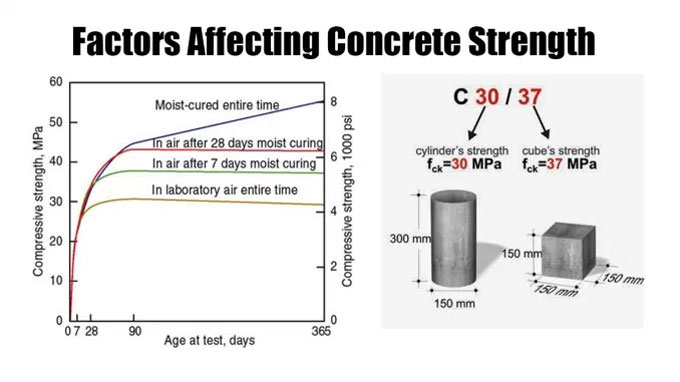# How concrete strength is impacted with different factorsThe strength of concrete is impacted by various factors. The details are given below :-

Concrete porosity: Air and water are the useful substances to fill up voids in concrete. Air voids belong to pores in concrete. When concrete is blended it contains air trapped in the mix. The vibrators are used to clear out the air at the time of pouring walls.

If the concrete is less porous, it’s strength will be increased and calculated with compressive strength. The most crucial source of porosity in concrete refers to the proportion of water to cement in the mix, called the ‘water to cement ratio’.

Factors water/cement ratio: It is described as the mass of water divided by the mass of cement in a mix. As for instance, in a concrete mix if there are 400kg cement and 240litres(=240kg) of water, the water/cement ratio will be 240/400=0.6. The water cement ratio is shortened as ‘w/c ratio’ or just ‘w/c’. In mixes where the w/c is in excess of roughly 0.4, all the cement can, in theory, react with water to develop cement hydration products. If the w/c ratios are greater, it follows that the space occupied by the supplementary water over w/c=0.4 will persist as pore space filled with water, or with air when the concrete becomes dry.

As a result, when the w/c ratio become higher, the porosity of the cement paste in the concrete also upsurges. With the higher porosity, the compressive strength of the concrete will reduce.

Stability of aggregate: It is inevitable that when the aggregate in concrete is feeble, the concrete also becomes feeble. Rocks like chalk that contain low intrinsic strength, are not appropriate to be utilized as aggregate.

Aggregate-paste bond: The strength of the bond among the paste and the aggregate is vital. When no bond exists, the aggregate practically reproduces a void and the strength of concrete is decreased.

Cement-related parameters: Various parameters pertaining to the formation of the individual cement minerals and their ratios in the cement can impact the rate of strength growth and the final strengths gained.